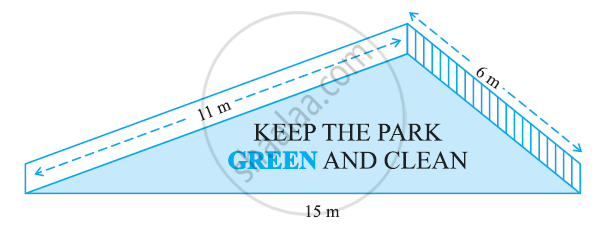# There is a slide in the park. One of its side walls has been painted in the same colour with a message “KEEP THE PARK GREEN AND CLEAN” (see the given figure). If the sides of the wall are 15m, 11m, and 6m, find the area painted in colour. - Mathematics

There is a slide in the park. One of its side walls has been painted in the same colour with a message “KEEP THE PARK GREEN AND CLEAN” (see the given figure). If the sides of the wall are 15m, 11m, and 6m, find the area painted in colour.#### Solution

It can be observed that the area to be painted in colour is a triangle, having its sides as 11 m, 6 m, and 15 m.

Perimeter of such a triangle = (11 + 6 + 15) m

s = 32 m

s = 16 m

By Heron’s formula,

"Area of Triangle "=sqrt(s(s-a)(s-b)(s-c))

= [sqrt(16(16-11)(16-6)(16-15))]m^2

=(sqrt(16xx5xx10xx1))m^2

=20sqrt2m^2

"Therefore, the area painted in colour is "20sqrt2m^2

Concept: Area of a Triangle by Heron's Formula
Is there an error in this question or solution?
Chapter 12: Heron's Formula - Exercise 12.1 [Page 203]

#### APPEARS IN

NCERT Class 9 Maths
Chapter 12 Heron's Formula
Exercise 12.1 | Q 3 | Page 203

Share